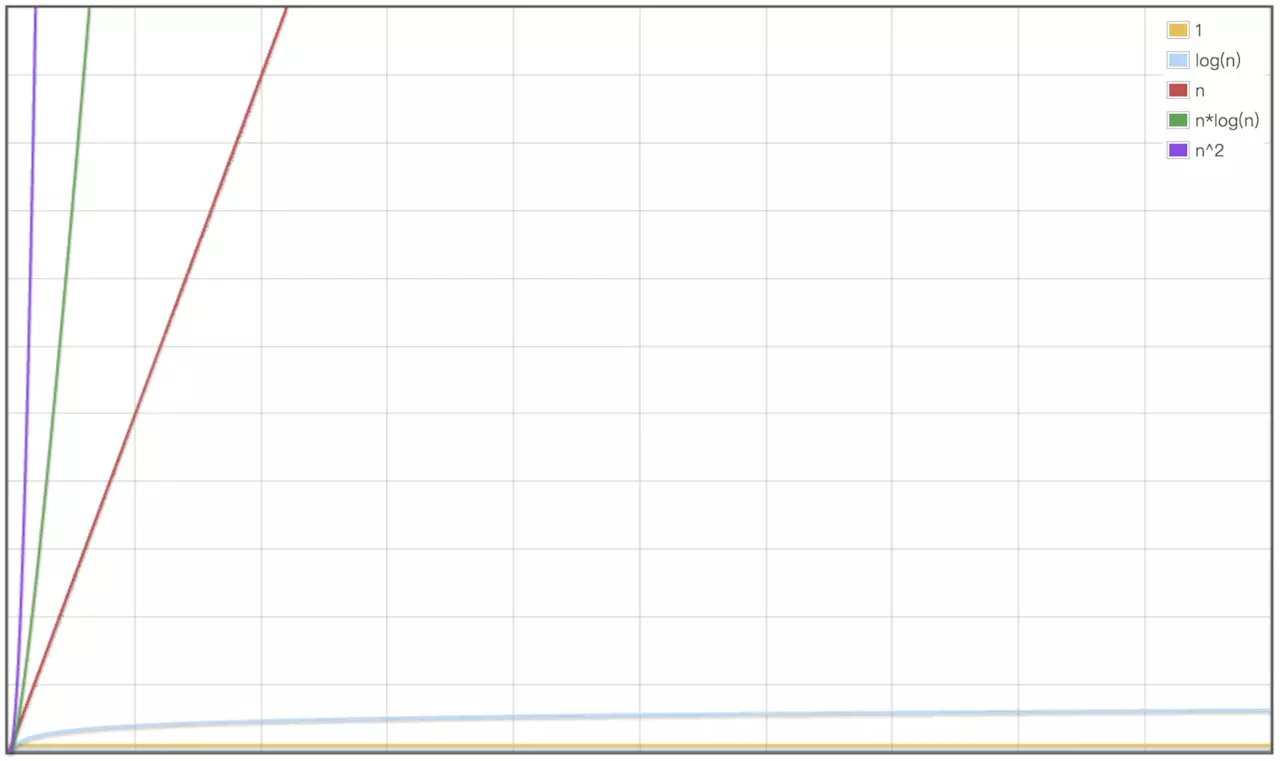前端轻松学算法：时间复杂度1. 为什么需要时间复杂度

2. 时间复杂度怎么表示

3. 怎样分析一段代码的时间复杂度

二、时间复杂度怎么表示

1 function factorial(){
2     let i = 0 // 执行了1次
3     let re = 1 // 执行了1次
4     for(;i < n; i++ ){ // 执行了n次
5         re*= n // 执行了n次
6     }
7     return re // 执行了1次
8 }

1 T(n) = 2t + 2nt + t = (2n ＋ 3)tT(n) = O(F(n))
• n: **代表数据规模, 相当于上面例子中的n

• F(n)：表示代码执行次数的总和，代码执行次数的总和与数据规模有关，所以用F(n)表示, F(n)对应上面例子中的（2n+3）

• T(n): 代表代码的执行时间，对应上面例子中的T(n)

• O: 大O用来表示代码执行时间T(n) 与 代码执行次数总和F（n）之间的正比关系。

1 T(n) = O(2n + 3)

1 T(n) = O(n)

1 function reverse(arr){
2     let n = arr.length // 执行了1次
3     let i = 0 // 执行了1次
4     for(;i<n-1;i++){ // 执行了n次
5         let j = 0 // 执行了n次
6         for(j=0;j<n-1;j++){ // 执行了n²次
7             var temp=arr[j] // 执行了n²次
8             arr[j]=arr[j+1] // 执行了n²次
9             arr[j+1]=temp // 执行了n²次
10         }
11     }
12 }

1 T(n) = (4n² + 2n + 2)t

1 T(n) = O(n²)

1. 不保留系数

2. 只保留最高阶

3. 嵌套代码的复杂度内外代码复杂度的乘积

三、如何快速分析一段代码的时间复杂度

1 function test(n){
2     let res = 0
3     if(n > 100){
4         const a = 1
5         const b = 100
6         res = (a + b)*100/2
7     }else {
8         let i = 1
9         for(;i<n; i++){
10             res+=i
11         }
12     }
13     return res
14 }

1 T(n) = O(1)

1 T(n) = O(n)

四、常见的时间复杂度1 O(1) < O(logn) < O(n) < O(nlogn) < O(n^2)

1 function binarySearch(arr,key){
2     const n = arr.length
3     let low = 0
4     let high = n - 1
5     let mid = Math.floor((low + high) / 2)
6     while (low <= high) {
7         mid = Math.floor((low + high) / 2)
8         if (key === arr[mid]) {
9             return mid
10         } else if (key < arr[mid]) {
11             high = mid - 1
12         } else {
13             low = mid + 1
14         }
15     }
16     return -1
17 }

... ...

如果喜欢本文请扫描下方二维码关注公众号。更多系列原创文章，就在「前端小苑」。posted @ 2019-04-28 18:31  wolfSoul  阅读(904)  评论(1编辑  收藏  举报